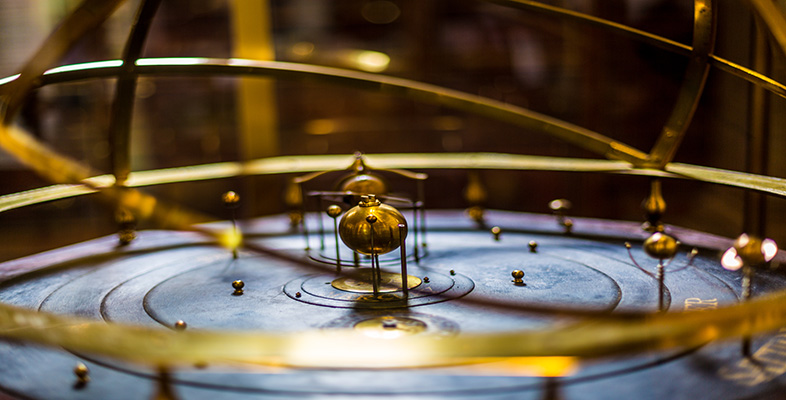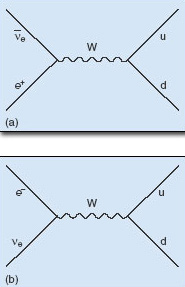Science, Maths & Technology

### Become an OU studentThe evolving Universe

Start this free course now. Just create an account and sign in. Enrol and complete the course for a free statement of participation or digital badge if available.

# 1.7 The hadron era

Time: 10−5 s to 100 s

Temperature: 3 × 1012 K to 109 K

Energy: 1 GeV to 300 keV

From the time that the temperature fell to about 3 × 1012 K, at about 10−5 s after the Big Bang, stable baryons (protons and neutrons) began to form from the up and down quarks that remained after the annihilation of matter and antimatter.

## Figure 10

How does the mean energy per particle at 10−5 s compare with the mass energy of a proton or neutron?

Protons and neutrons have a mass energy of about 1 GeV, which is similar to the mean energy per particle in the Universe at this time.

This is why confinement of quarks became important from this time onwards. Before 10−5 s after the Big Bang, there had been sufficient energy available for up and down quarks to escape to distances significantly larger than the dimensions of a proton or neutron. After this time, no such escape was possible.

## Question 11

What are the quark contents of a proton and a neutron?

A proton is composed of two up quarks and a down quark, whereas a neutron is composed of two down quarks and an up quark.

Equal numbers of up and down quarks therefore led to an equal number of protons and neutrons emerging from this process. To recap on the contents of the Universe at this time, there were about a billion photons, electrons, positrons, neutrinos and antineutrinos for every single proton or neutron in the Universe.

## Question 12

Why had the electrons and positrons not yet mutually annihilated each other?

The mass energy of an electron or positron is about 500 keV, and the mean energy per particle was still much higher than the 1 MeV required to create a pair of them. So electrons and positrons were still in equilibrium with photons, undergoing both annihilation and pair creation reactions at the same rate.

As soon as baryons had formed, weak interactions took over, with protons and neutrons existing in equilibrium governed by the following processes:

So neutrons converted into protons by reacting with either positrons or electron neutrinos; protons converted into neutrons by reacting with either electron antineutrinos or electrons.

## Question 13

At the quark and lepton level, how may the two reactions in Equation 3 be represented?

Bearing in mind the quark composition of a proton and a neutron, each of the reactions involve conversions between a down quark and an up quark as shown in Equations 4a and b:

We can also draw Feynman diagrams to illustrate these two processes, as shown in Figure 5. Each of the two processes may be considered as arising from the exchange of a W boson. Furthermore, each of the parts of this figure may be read either from bottom to top, or from top to bottom, depending on which way the reaction in Equation 4 progresses.

## Question 14

What is the change in electric charge when a positron converts into an electron antineutrino? What is the change in electric charge when a down quark converts into an up quark? How can the exchange of a W boson, as illustrated in Figure 5a, maintain conservation of electric charge in this case?